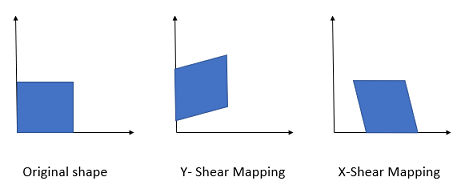# Shear Mapping: Definition, Examples

Shear mapping is a special kind of linear mapping. Points on one axis remain unchanged, while all other points are shifted parallel to that axis.• Each point moves a distance which is proportional to their (signed) perpendicular distance from the axis,
• All points on one side of the stationary axis are transformed in the same direction,
• Areas are always transformed into equal areas.

## Example of a Shear Mapping

One example of a shear mapping is the mapping which sends all points (x, y) to (x + 3y, y). In this case the x axis will remain unchanged. Every point is transformed by a distance that is proportional to the signed distance from the x axis. Points on either side of the x axis will be transformed in opposite directions.

Since every point is moved horizontally, we call this mapping a horizontal shear.

## Horizontal and Vertical Shear Mapping

In general, a shear mapping will transform a point (x, y) to (x + ay, y + bx). But the easiest mappings to understand are those for which one of the Cartesian axes is stationary: Horizontal and Vertical Shears.

Horizontal shears, like the transformation in our example above, transform a point with coordinates (x, y) to the point (x + my, y). A vertical shear, similarly, transforms every point (x, y) to the point (x, y + mx).

In these equations m represents a fixed parameter specific to the mapping; we call it the shear factor.

For a horizontal shear, vertical lines that are not identical to the x axis become oblique lines with slope 1/m. The image below shows a horizontal shear in the plane; the shear factor is 1.25. The original grid is in green, and the black dot represents the origin. The green shadow represents the result of the mapping.